14.4 自组织图¶

$m_j$ 的邻居定义为使得 $\ell_j$ 和 $\ell_k$ 间的距离小的所有 $m_k$．最简单的方式是采用欧式距离，通过阈值 $r$ 来决定“小”．邻域经常包含最近的原型 $m_j$ 本身．

weiya 注：

SOM 算法的效果取决于学习速率 $\alpha$ 和距离阈值 $r$．一般地，$\alpha$ 随着迭代的进行 $\alpha$ 从 1.0 下降至 0.0．类似地，随着迭代的进行，$r$ 从初始值 $R$ 线性降至 1．我们将在下面的例子中阐释一种用来选择 $R$ 的方法．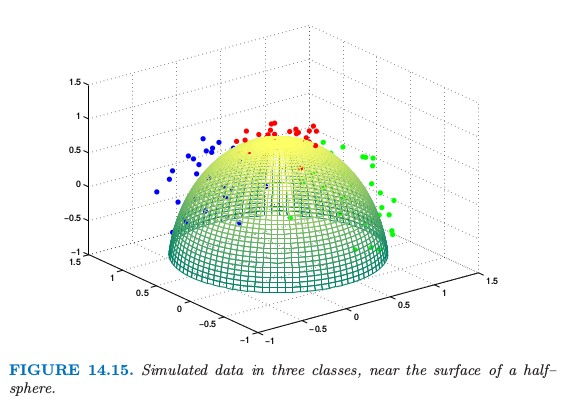weiya 注：$R = 2$

• 四个顶点，$(1, 1), (1, 5), (5, 1), (5,5)$: 6 个
• 中心点，$(3, 3)$: 13 个
• $(2, 3)$: 12 个
• $(1, 3)$: 9 个
• ....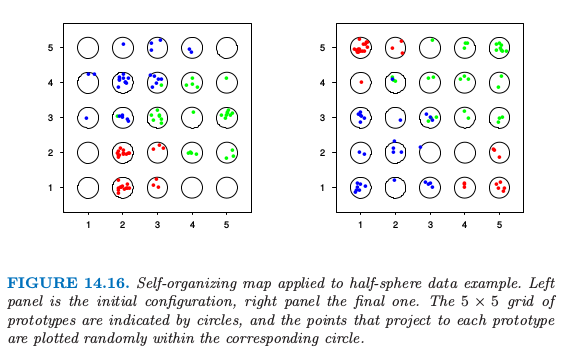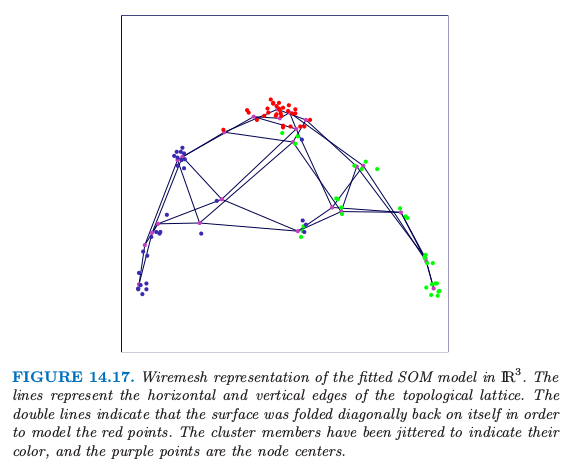weiya 注：Ex. 14.5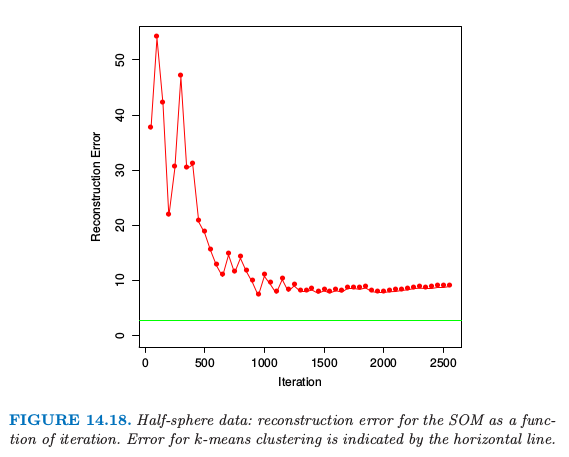例子：文件组织与检索¶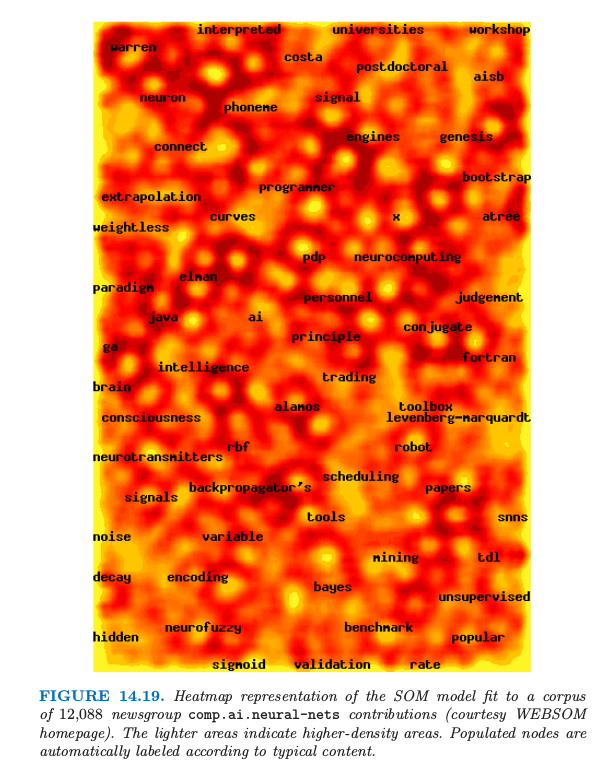weiya 注

term-document 矩阵示意图如下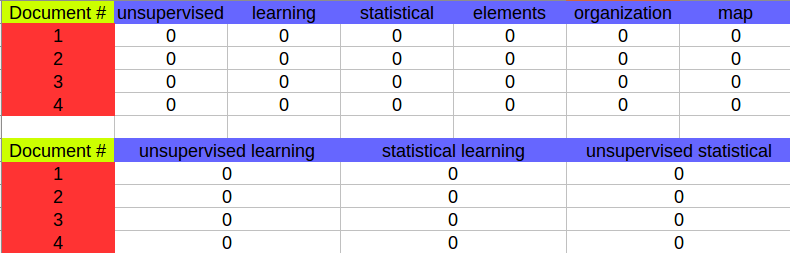图中第一个每一行的值是每个单词的相对频率，而第二个每一行的值是双字母组的相对频率．

1. Kohonen, T., Kaski, S., Lagus, K., Saloj¨arvi, J., Paatero, A. and Saarela, A. (2000). Self-organization of a massive document collection, IEEE Transactions on Neural Networks 11(3): 574–585. Special Issue on Neural Networks for Data Mining and Knowledge Discovery.